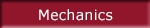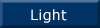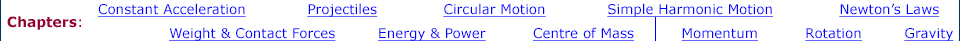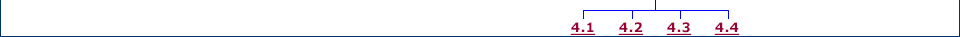Links to related material and teachers' resources Background material for simple harmonic motion SHM as a projection of circular motion. Displacement, velocity and acceleration, Chladni patterns and sinusoidal vibration. Oscillationsis the multimedia chapter on Oscillations, which analyses the forces and energies involved in Simple Harmonic Motion. Background to Oscillations The physics of oscillation, forces and energy, the pendulum, damped oscillations, forced oscillations, resonance in 1, 2 and 3 dimensions. More about chladni patterns Modes of vibration, nodes, naming of modes, examples on homogeneous plates and violin plate. Phasor addition Phasor representation of Simple Harmonic Motion. Adding phasors with different amplitudes and phase but equal frequency. Constructive and destructive interference. What if frequencies are different? Phasor and Lissajous representations compared. AC circuits Resisance, reactance and impedance. Capacitors and charging, inductors and the Faraday emf. Phasor sums in circuits. Resonance, bandwidth and Q factor, complex impedance. What is a sound spectrum? Summing sine waves to produce a complex waveform, harmonics, Fourier synthesis. Download all animations in this chapter (or individually below each thumbnail) Simple harmonic motion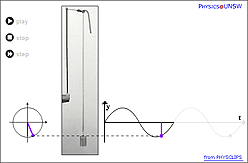Download (.zip) Download (.zip)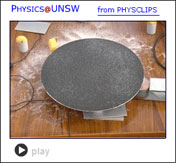Download (.zip) Download (.zip) Download (.zip)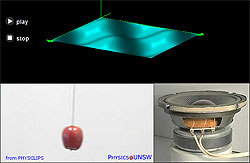Download (.zip) Download (.zip)